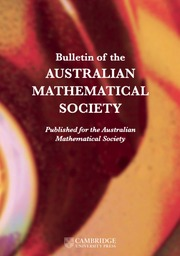Home
Hostname: page-component-7ccbd9845f-6pjjk Total loading time: 0.843 Render date: 2023-02-01T04:26:05.680Z Has data issue: true Feature Flags: { "useRatesEcommerce": false } hasContentIssue trueBulletin of the Australian Mathematical Society

## Abstract

Let$[t]$ be the integral part of the real number t and let$\mathbb {1}_{{\mathbb P}}$ be the characteristic function of the primes. Denote by$\pi _{\mathcal {S}}(x)$ the number of primes in the floor function set$\mathcal {S}(x) := \{[{x}/{n}] : 1\leqslant n\leqslant x\}$ and by$S_{\mathbb {1}_{{\mathbb P}}}(x)$ the number of primes in the sequence$\{[{x}/{n}]\}_{n\geqslant 1}$ . Improving a result of Heyman [‘Primes in floor function sets’, Integers22 (2022), Article no. A59], we show\begin{align*} \pi_{\mathcal{S}}(x) = \int_2^{\sqrt{x}} \frac{d t}{\log t} + \int_2^{\sqrt{x}} \frac{d t}{\log(x/t)} + O(\sqrt{x}\,\mathrm{e}^{-c(\log x)^{3/5}(\log\log x)^{-1/5}}) \quad\mbox{and}\quad S_{\mathbb{1}_{{\mathbb P}}}(x) = C_{\mathbb{1}_{{\mathbb P}}} x + O_{\varepsilon}(x^{9/19+\varepsilon}) \end{align*}

for$x\to \infty$ , where$C_{\mathbb {1}_{{\mathbb P}}} := \sum _{p} {1}/{p(p+1)}$ ,$c>0$ is a positive constant and$\varepsilon$ is an arbitrarily small positive number.

## MSC classification

Type
Research Article
© The Author(s), 2022. Published by Cambridge University Press on behalf of Australian Mathematical Publishing Association Inc.

## Access options

Get access to the full version of this content by using one of the access options below. (Log in options will check for institutional or personal access. Content may require purchase if you do not have access.)

## Footnotes

This work is supported in part by the National Natural Science Foundation of China (Grant Nos. 11971370 and 12071375).

## References

Bordellès, O., ‘On certain sums of number theory’, Int. J. Number Theory 18(99) (2022), 20532074.CrossRefGoogle Scholar
Bordellès, O., Dai, L., Heyman, R., Pan, H. and Shparlinski, I. E., ‘On a sum involving the Euler function’, J. Number Theory 202 (2019), 278297.CrossRefGoogle Scholar
Davenport, H., Multiplicative Number Theory, 3rd edn, Graduate Texts in Mathematics, 74 (Springer-Verlag, New York, 2000), revised and with a preface by H. L. Montgomery.Google Scholar
Friedlander, J. and Iwaniec, H., ‘The polynomial${X}^2+{Y}^4$ captures its primes’, Ann. of Math. (2) 148(3) (1998), 9451040.CrossRefGoogle Scholar
Heath-Brown, D. R., ‘Primes represented by${x}^3+2{y}^3$ ’, Acta Math. 186(1) (2001), 184.CrossRefGoogle Scholar
Heyman, R., ‘Cardinality of a floor function set’, Integers 19 (2019), Article no. A67.Google Scholar
Heyman, R., ‘Primes in floor function sets’, Integers 22 (2022), Article no. A59.Google Scholar
Huxley, M. N., ‘On the difference between consecutive primes’, Invent. Math. 15 (1972), 164170.CrossRefGoogle Scholar
Liu, K., Wu, J. and Yang, Z.-S., ‘On some sums involving the integral part function’, Preprint, 2021, arXiv:2109.01382v1.Google Scholar
Liu, K., Wu, J. and Yang, Z.-S., ‘A variant of the prime number theorem’, Indag. Math. (N.S.) 33 (2022), 388396.CrossRefGoogle Scholar
Ma, J. and Wu, J., ‘On a sum involving the von Mangoldt function’, Period. Math. Hungar. 83(1) (2021), 3948.CrossRefGoogle Scholar
Rivat, J. and Sargos, P., ‘Nombres premiers de la forme$\left[{n}^c\right]$ ’, Canad. J. Math. 53(2) (2001), 414433 (in French). English summary.CrossRefGoogle Scholar
Wu, J., ‘Note on a paper by Bordellès, Dai, Heyman, Pan and Shparlinski’, Period. Math. Hungar. 80 (2020), 95102.CrossRefGoogle Scholar
Yu, Y. and Wu, J., ‘Distribution of elements of a floor function set in arithmetical progression’, Bull. Aust. Math. Soc. 106(3) (2022), 419424.Google Scholar
Zhai, W., ‘On a sum involving the Euler function’, J. Number Theory 211 (2020), 199219.CrossRefGoogle Scholar
1
Cited by

# Save article to Kindle

Note you can select to save to either the @free.kindle.com or @kindle.com variations. ‘@free.kindle.com’ emails are free but can only be saved to your device when it is connected to wi-fi. ‘@kindle.com’ emails can be delivered even when you are not connected to wi-fi, but note that service fees apply.

Find out more about the Kindle Personal Document Service.

ON THE PRIMES IN FLOOR FUNCTION SETS
Available formats
×

# Save article to Dropbox

To save this article to your Dropbox account, please select one or more formats and confirm that you agree to abide by our usage policies. If this is the first time you used this feature, you will be asked to authorise Cambridge Core to connect with your Dropbox account. Find out more about saving content to Dropbox.

ON THE PRIMES IN FLOOR FUNCTION SETS
Available formats
×

# Save article to Google Drive

To save this article to your Google Drive account, please select one or more formats and confirm that you agree to abide by our usage policies. If this is the first time you used this feature, you will be asked to authorise Cambridge Core to connect with your Google Drive account. Find out more about saving content to Google Drive.

ON THE PRIMES IN FLOOR FUNCTION SETS
Available formats
×
×